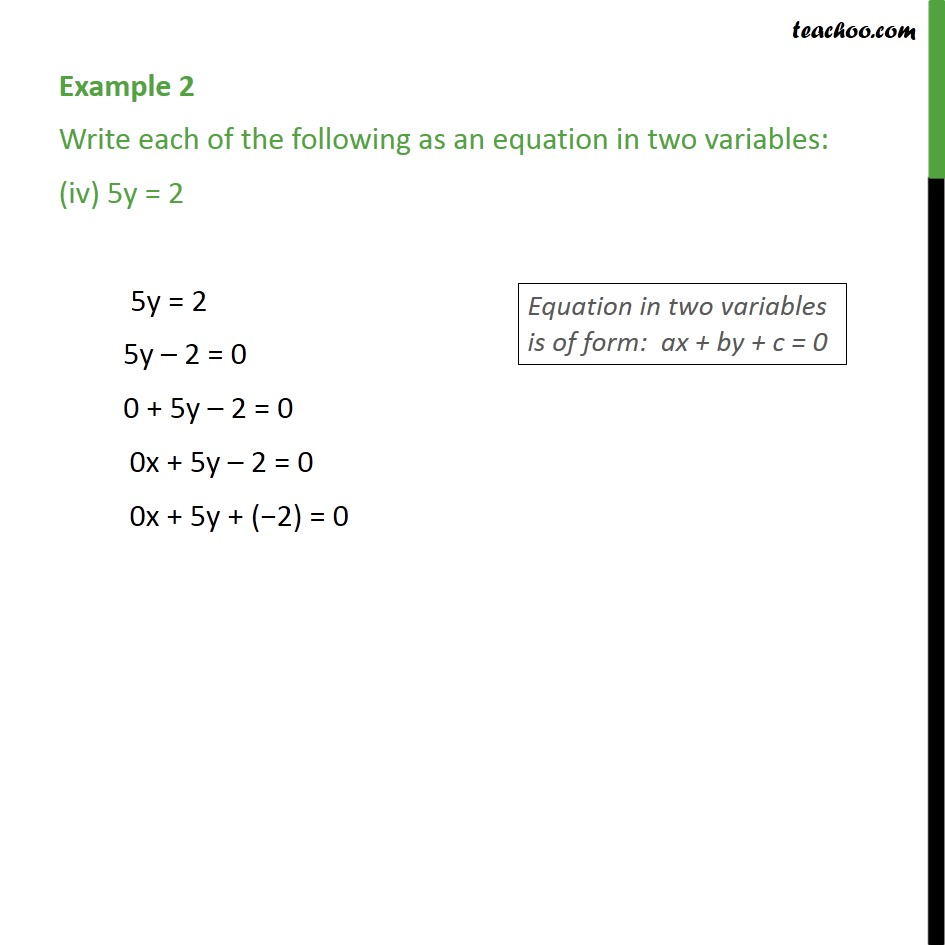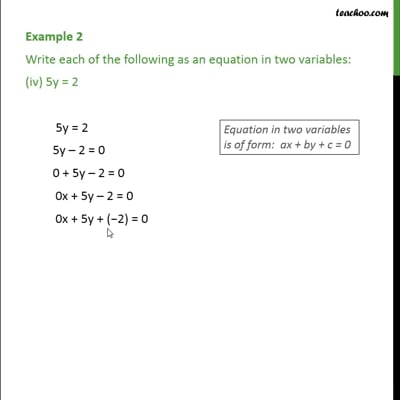Examples

Chapter 4 Class 9 Linear Equations in Two Variables
Serial order wiseThis video is only available for Teachoo black users

Get live Maths 1-on-1 Classs - Class 6 to 12

### Transcript

Example 2 Write each of the following as an equation in two variables: (iv) 5y = 2 5y = 2 5y 2 = 0 0 + 5y 2 = 0 0x + 5y 2 = 0 0x + 5y + ( 2) = 0